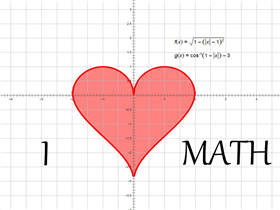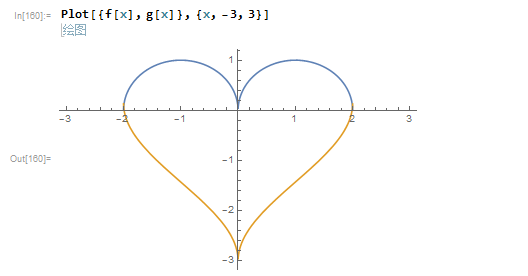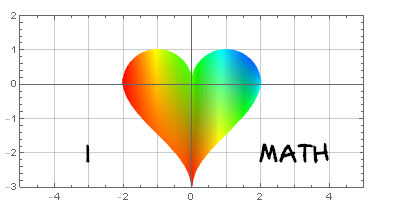# Mathematica绘制’I LOVE MATH’|心型函数的绘制

• A+## 心型函数

``````
f[x_] := Sqrt[1 - (Abs[x] - 1)^2]

g[x_] := ArcCos[1 - Abs[x]] - 3
``````## 美化图片

``````
name = "Axure Handwriting";

p1 = Plot[{f[x], g[x]}, {x, -3, 3},

PlotRange -> {{-5, 5}, {-3, 2}},

Frame -> True, GridLines -> {Range[-4, 4], Range[-3, 1]},

AspectRatio -> .5,

ColorFunction -> Function[{x, y}, Hue[x*.7 y]],

Filling -> {1 -> {2}}];

p2 = Graphics[{

Text[Style["I", Bold, 25, FontFamily -> name], {-3, -2}],

Text[Style["MATH", Bold, 25, FontFamily -> name], {3, -2}]

}];

Show[p1, p2]
``````• 微信公众号
• 关注微信公众号
•• QQ群
• 我们的QQ群号
•